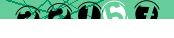# Sorting SimpleXMLElement Object arrays

How annoying is it when you import data from an XML file (using simplexml_load_string for example) and get what looks like a normal array, but turns out to be a SimpleXMLElement Object which doesn't accept most of the usual array functions.

## Sorting SimpleXMLElement Objects

After importing an XML file into PHP we find ourselves with the following structure. The original XML being a series of <result> nodes under a single parent node. A very common structure.

At this stage we can see the data using print_r or similar function which can loop through the SimpleXMLElement Object structure:

```SimpleXMLElement Object ( [result] => Array (  => SimpleXMLElement Object ( [zip] => 90210 [city] => Los Angeles [state] => CA )  => SimpleXMLElement Object ( [zip] => 90213 [city] => Beverly Hills [state] => CA )  => SimpleXMLElement Object ( [zip] => 92130 [city] => San Diego [state] => CA )  => SimpleXMLElement Object ( [zip] => 89165 [city] => Las Vegas [state] => NV )  => SimpleXMLElement Object ( [zip] => 85034 [city] => Phoenix [state] => AZ ) ) ) ```

While it looks like \$xml->result might be a normal array, trying to apply the usual array functions will not work. Even var_dump will not show you past the first entry as the data for SimpleXMLElement Objects is stored internally by PHP.

## Creating a proxy array for sorting

What we can do, however, is create a 'proxy' array for sorting by looping through the results and collecting each node:

```<?PHP \$sortable = array(); foreach(\$xml->result as \$node) { \$sortable[] = \$node; } ?>```

Now we have a normal PHP Array containing SimpleXMLElement Object nodes:

```Array (  => SimpleXMLElement Object ( [zip] => 90210 [city] => Los Angeles [state] => CA )  => SimpleXMLElement Object ( [zip] => 90213 [city] => Beverly Hills [state] => CA )  => SimpleXMLElement Object ( [zip] => 92130 [city] => San Diego [state] => CA )  => SimpleXMLElement Object ( [zip] => 89165 [city] => Las Vegas [state] => NV )  => SimpleXMLElement Object ( [zip] => 85034 [city] => Phoenix [state] => AZ ) ) ```

The reason for PHP keeping SimpleXmlElement data in memory is that it can become very large in which case extracting it into other PHP constructs will consume a lot of memory. This option is best then for small datasets.

## Applying the sort

Our Array can now be sorted using a variation of our associative array sorting functions. For example, to sort the list by state and then by city we use the following:

```<PHP function compare_city(\$a, \$b) { // sort by state \$retval = strnatcmp(\$a->state, \$b->state); // if identical, sort by city if(!\$retval) \$retval = strnatcmp(\$a->city, \$b->city); return \$retval; } // sort alphabetically by state and city usort(\$sortable, __NAMESPACE__ . '\compare_city'); ?>```

The only real difference between this case and a normal associative array sorting function is that we reference the node elements using \$object->city instead of \$array['city']. The result is as you would expect:

```Array (  => SimpleXMLElement Object ( [zip] => 85034 [city] => Phoenix [state] => AZ )  => SimpleXMLElement Object ( [zip] => 90213 [city] => Beverly Hills [state] => CA )  => SimpleXMLElement Object ( [zip] => 90210 [city] => Los Angeles [state] => CA )  => SimpleXMLElement Object ( [zip] => 92130 [city] => San Diego [state] => CA )  => SimpleXMLElement Object ( [zip] => 89165 [city] => Las Vegas [state] => NV ) ) ```

And we're there. Check out the Related Articles links below for more on sorting of arrays and associative arrays.

< PHP

#### Send a message to The Art of Web:<- copy the digits from the image into this box

press <Esc> or click outside this box to close